# Faceting with `ggplot2`

This post describes all the available options to use small multiples with R and ggplot2. it shows how to efficiently split the chart window by row, column or both to show every group of the dataset separately.

# What is small multiple

Faceting is the process that split the chart window in several small parts (a grid), and display a similar chart in each section. Each section usually shows the same graph for a specific group of the dataset. The result is usually called small multiple.

It is a very powerful technique in data visualization, and a major advantage of using `ggplot2` is that it offers functions allowing to build it in a single line of code. Here is an example showing the evolution of a few baby names in the US. (source)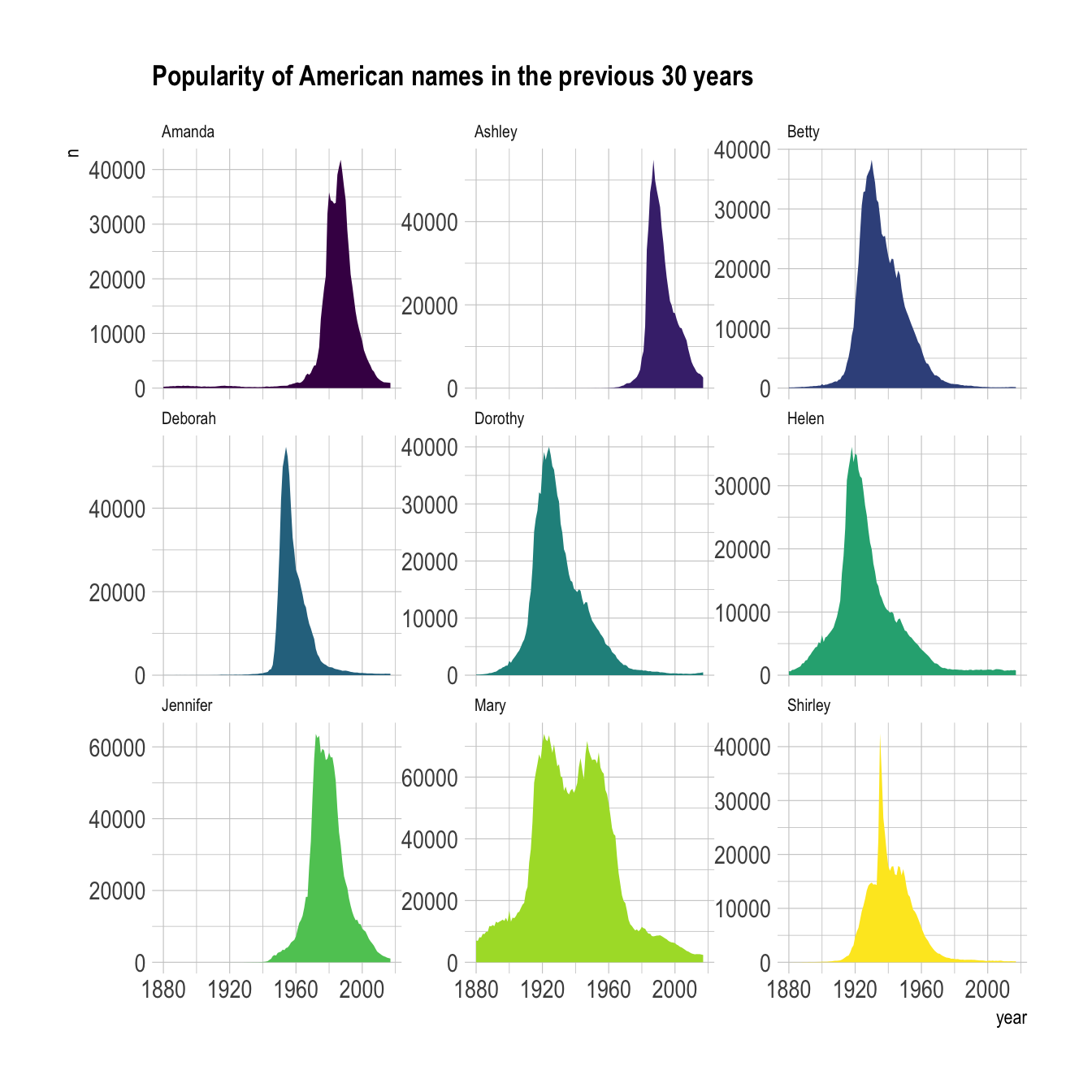``````# Libraries
library(tidyverse)
library(hrbrthemes)
library(babynames)
library(viridis)

data\$date <- as.Date(data\$date)

don <- babynames %>%
filter(name %in% c("Ashley", "Amanda", "Mary", "Deborah",   "Dorothy", "Betty", "Helen", "Jennifer", "Shirley")) %>%
filter(sex=="F")

# Plot
don %>%
ggplot( aes(x=year, y=n, group=name, fill=name)) +
geom_area() +
scale_fill_viridis(discrete = TRUE) +
theme(legend.position="none") +
ggtitle("Popularity of American names in the previous 30 years") +
theme_ipsum() +
theme(
legend.position="none",
panel.spacing = unit(0, "lines"),
strip.text.x = element_text(size = 8),
plot.title = element_text(size=13)
) +
facet_wrap(~name, scale="free_y")``````

# Faceting with `facet_wrap()`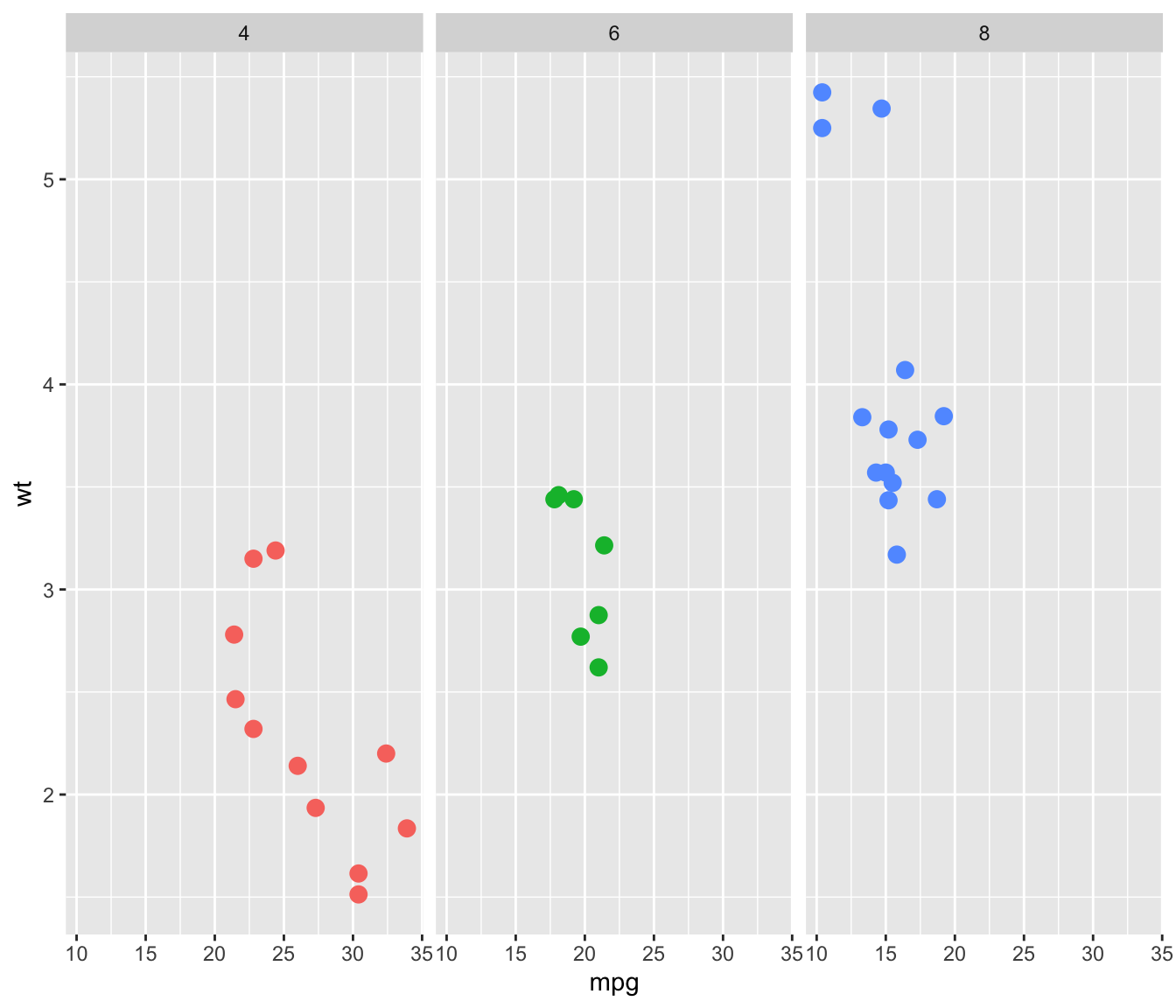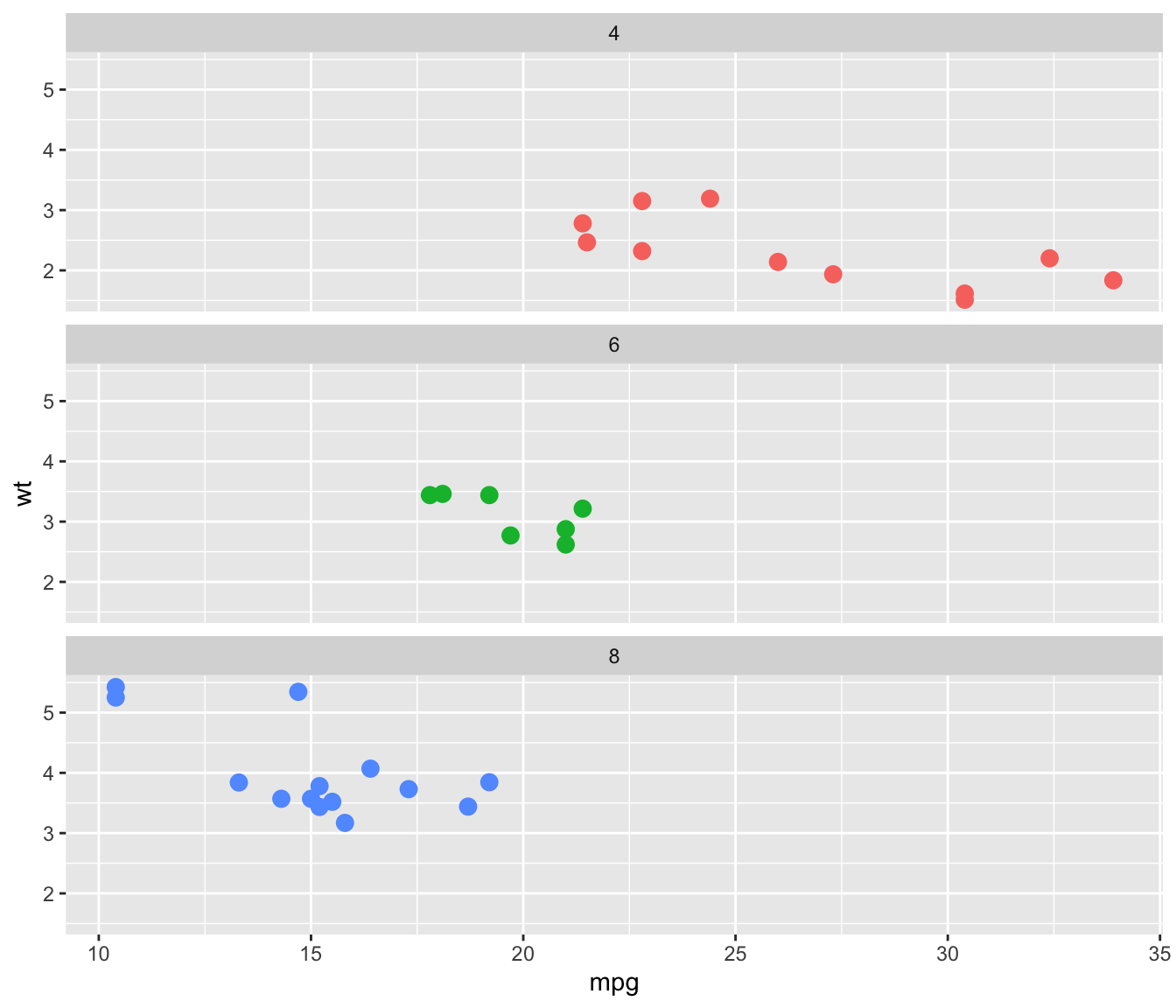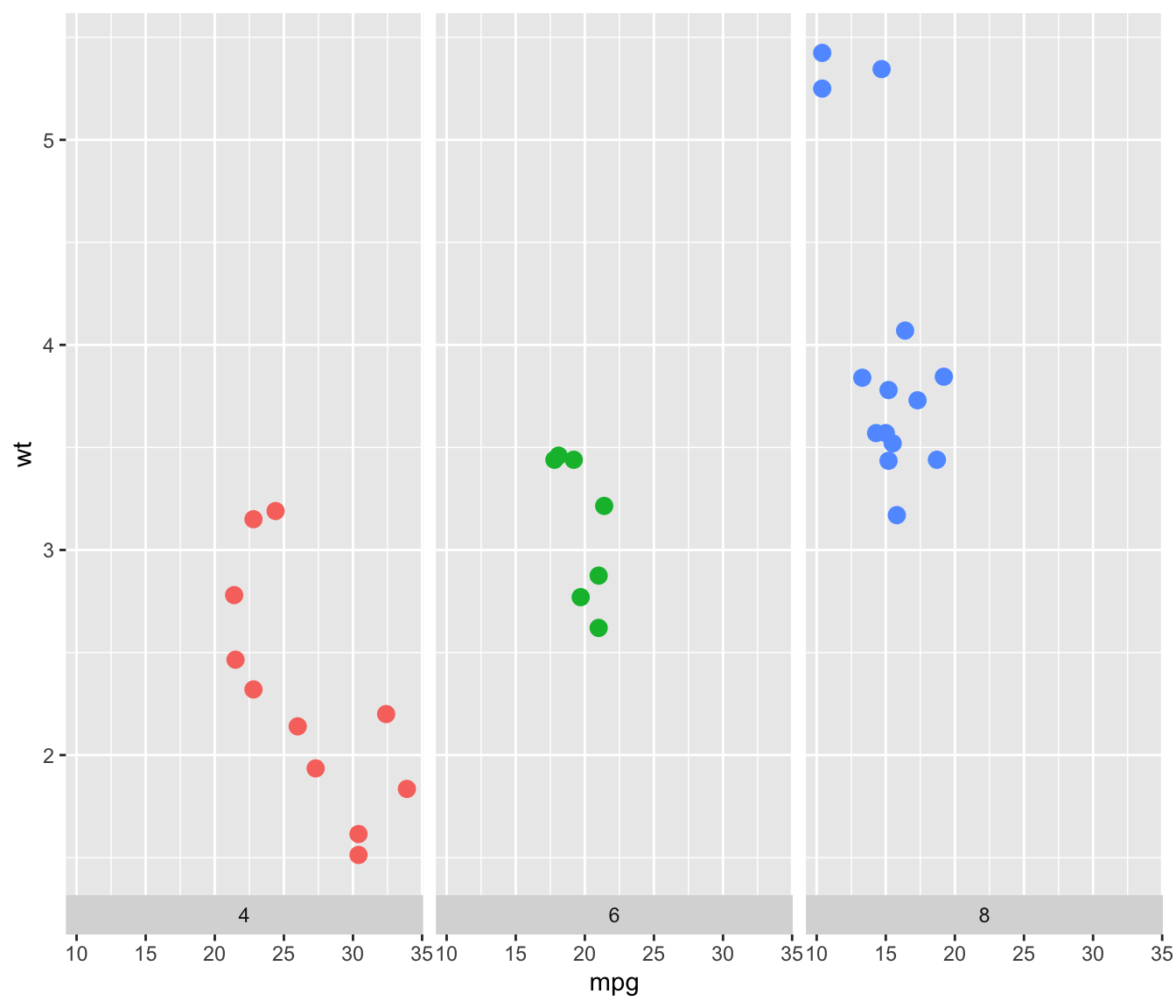`facet_wrap()` is the most common function for faceting with `ggplot2`. It builds a new chart for each level of a categorical variable. You can add the charts horizontally (graph1) or vertically (graph2, using `dir="v"`). Note that if the number of group is big enough, `ggplot2` will automatically display charts on several rows/columns.

The grey bar showing the related level can be placed on top or on the bottom thanks to the `strip.position` option (graph3).

Last but not least, you can choose whether every graph have the same scale or not with the `scales` option (graph4).

``````# library & datset
library(ggplot2)

# Split by columns (default)
ggplot( mtcars , aes(x=mpg, y=wt, color=as.factor(cyl) )) +
geom_point(size=3) +
facet_wrap(~cyl) +
theme(legend.position="none")``````
``````# Split by row
ggplot( mtcars , aes(x=mpg, y=wt, color=as.factor(cyl)  )) +
geom_point(size=3) +
facet_wrap(~cyl , dir="v")  +
theme(legend.position="none")``````
``````# Add label at the bottom
ggplot( mtcars , aes(x=mpg, y=wt, color=as.factor(cyl)  )) +
geom_point(size=3) +
facet_wrap(~cyl , strip.position="bottom") +
theme(legend.position="none")``````
``````# Use same scales for all
ggplot( mtcars , aes(x=mpg, y=wt, color=as.factor(cyl)  )) +
geom_point(size=3) +
facet_wrap(~cyl , scales="free" ) +
theme(legend.position="none")``````

# `facet_grid()`

`facet_grid()` is the second function allowing to build small multiples with `ggplot2`. It allows to build one chart for each combinations of 2 categorical variables. One variable will be used for rows, the other for columns.

The two variables must be given around a `~`, the first being displayed as row, the second as column. The `facet-grid()` function also accepts the `scales` argument described above.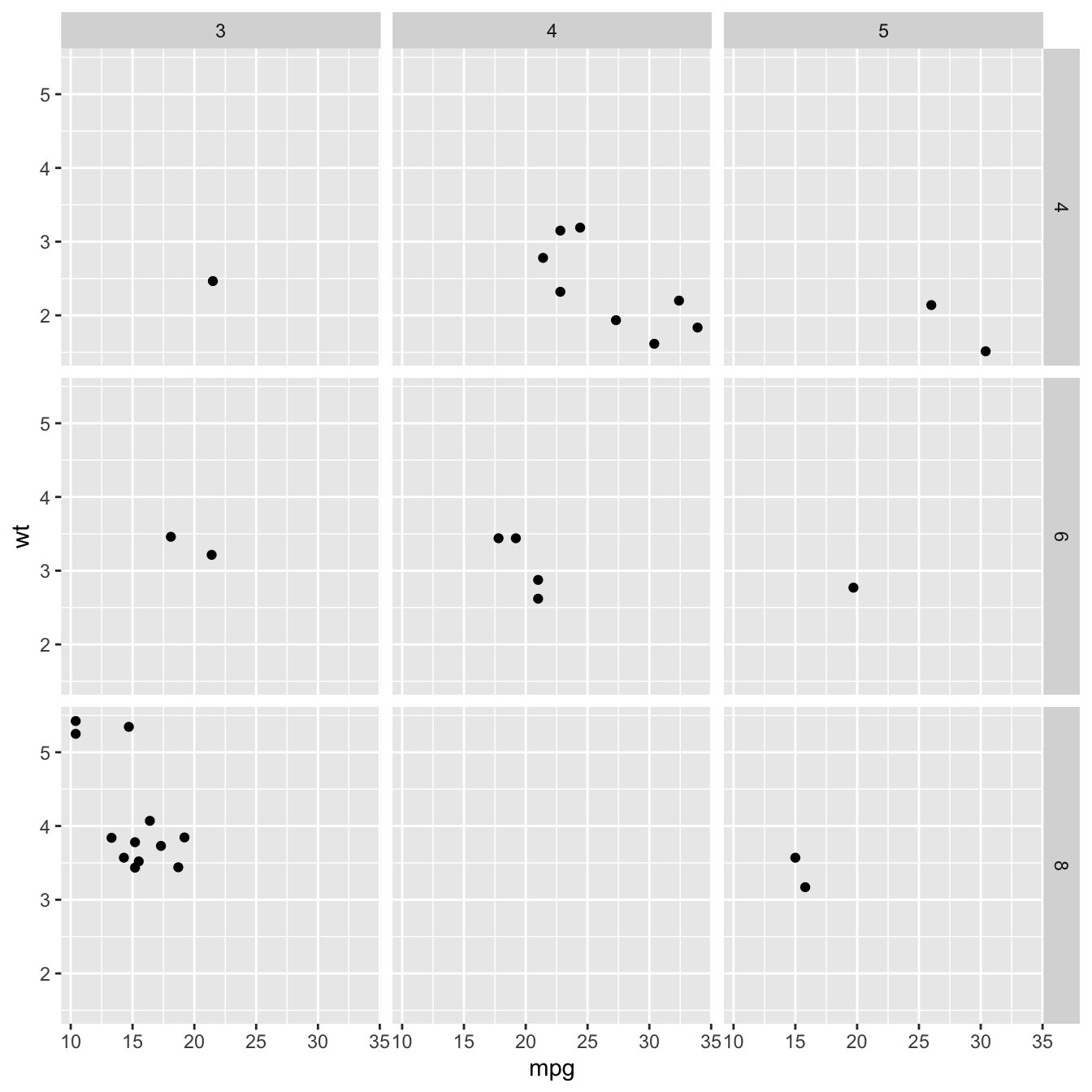``````# Faceted ggplot2 using facet_grid():
ggplot( mtcars , aes(x=mpg, y=wt )) +
geom_point() +
facet_grid( cyl ~ gear)``````

Related chart types

## Contact

This document is a work by Yan Holtz. Any feedback is highly encouraged. You can fill an issue on Github, drop me a message on Twitter, or send an email pasting yan.holtz.data with gmail.com.